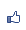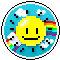唐老鴨之家 市長：燢 　副市長： 加入本城市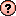｜推薦本城市｜加入我的最愛｜訂閱最新文章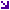本城市首頁討論區精華區投票區影像館推薦連結公告區訪客簿市政中心 (0)動態霍夫曼編碼Ｃ語言程式 瀏覽1,841｜回應0｜推薦0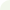燢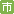等級：6 留言｜加入好友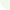/* * * * * * * * * * * * * * * * * * * * * * * * * * * * * * * * *
* DYNAMIC HUFFMAN CODING PROGRAM (huffman.c)
* * * * * * * * * * * * * * * * * * * * * * * * * * * * * * * * */

#define MAXN 256
#include
#include "huffman.h"
int q;
main()
{
int i;
char code;
initialize();
scanf("%s",code);
for( i = 0; i < strlen(code); i ++ ){
putchar('\n');
putchar(code[i]);
putchar(' ');
encode( code[i] );
update( code[i] );
}
}
/**********************/
initialize()
{
int i;
M = 0;
E = 0;
R = -1;
Z = 2 * MAXN - 1;
for( i = 1; i <= MAXN; i ++ ){
M ++;
R ++;
if( 2 * R == M ){
E ++;
R = 0;
}
A[i] = i;
C[i] = i;
}
H = 1;
L[H] = H;
G[H] = H;
W[H] = 0;
A = Z;
D[H] = Z;
V = 2;
for( i = V; i <= Z - 1; i ++ )
G[i] = i + 1;
G[Z] = 0;
P[MAXN] = 0;
C[Z] = 0;
B[Z] = H;
}

encode( k )
int k;
{
int i, j, t;
i = 0;
q = A[k];
if( q <= M ){ /* encode zero weight */
q --;
if( q < 2 * R )
t = E + 1;
else{
q = q - R;
t = E;
}
for( j = 1; j <= t; j ++ ){
i ++;
S[i] = q % 2;
q = q / 2;
}
q = A;
}
while( q < Z ){
i ++;
S[i] = ( q + 1 ) % 2;
q = P[( q + 1) / 2];
}
while( i > 0 )
printf("%d", S[i--] );
}

update( k ) /* used by both sender and receiver
after ak has been encoded or decoded */
int k;
{
set_node( k ); /* set q to the external node whose weight
should increase */
while( q > 0 ){
move_right(); /* move q to the right of its block */
trans_high(); /* transfer q to the next block,
with weight one higher */
q = P[( q + 1 ) / 2];
}
}

set_node( k )
int k;
{
q = A[k];
if( q <= M ){ /* a zero weight will become positive */
A[C[M]] = q;
C[q] = C[M];
if( R == 0 ){
R = M / 2;
if( R > 0 )
E --;
}
M --;
R --;
if( M > 0 ){
q = A - 1;
A = q - 1;
A[k] = q;
C[q] = k;
if( M > 1 )
C[q-1] = 0;
P[M] = q + 1;
C[q + 1] = M;
B[q] = H;
B[q - 1] = H;
}
}
}

move_right()
{
int t, ct, cq, acq;
if( q < D[B[q]] && D[B[P[( q + 1 ) / 2]]] > q + 1){
/* exchange two subtrees of the same weight,
assuming that neither is the child of the other */
t = D[B[q]];
ct = C[t];
cq = C[q];
acq = A[cq];
if( A[ct] != t )
P[ct] = q;
else
A[ct] = q;
if( acq != q )
P[cq] = t;
else
A[cq] = t;
C[t] = cq;
C[q] = ct;
q = D[B[q]];
}
}

trans_high()
{
int j, u, gu, lu, x, t, qq;
u = B[q];
gu = G[u];
lu = L[u];
x = W[u];
qq = D[u];
if( W[gu] == x + 1 ){
B[q] = gu;
B[qq] = gu;
if( D[lu] == q - 1 || ( u == H && q == A )){
/* block u disappears */
G[lu] = gu;
L[gu] = lu;
if( H == u )
H = gu;
G[u] = V;
V = u;
}
else
D[u] = q - 1;
}
else{
if( D[lu] == q - 1 || ( u == H && q == A ) )
W[u] = x + 1;
else{ /* a new block appears */
t = V;
V = G[V];
L[t] = u;
G[t] = gu;
L[gu] = t;
G[u] = t;
W[t] = x + 1;
D[t] = D[u];
D[u] = q - 1;
B[q] = t;
B[qq] = t;
}
}
q = qq;
}

/* * * * * * * * * * * * * * * * * * * * * * * * * * * * * * * * *
* DYNAMIC HUFFMAN CODING DECLARATION FILE (huffman.h)
* * * * * * * * * * * * * * * * * * * * * * * * * * * * * * * * */

int S[MAXN];
/* a stack that holds bits before they are transmitted */
int M,E,R;
/* zero-weight counters, the number of zero-weight elements
is M=2^E+R, where 0<=R<2^E, except that M=0 implies R=-1 */
int P[MAXN+1];
/* pointer to the parents of nodes, the parents of nodes 2j-1
and 2j is node P[j] */
int C[2*MAXN];
/* pointer to the children of nodes, if node k is internal,
and if its children are node 2j-1 and 2j, then C[k]=j,
while if node k is external, it represents letter aC[k] */
int A[MAXN+2];
/* representation of the alphabet, if letter ak is currently
the jth letter of weight 0, then A[k]=j<=M, otherwise A[k]
is the number of the external node corresponding to ak */
int B[2*MAXN];
/* pointer to the blocks of nodes, all nodes j of a given
weight have the same value of B[j] */
int W[2*MAXN];
/* the weights, block k has weight W[k] */
int L[2*MAXN];
/* left pointer for blocks, the nearest block whose weight
is less than that of block k is block L[k], unless
block k has the smallest weight, in which case L[k] is
the block of largest weight */
int G[2*MAXN];
/* right pointer for blocks, the nearest block whose weight
is greater than that of block k is block G[k], unless
block k has the greatest weight, in which case G[k] is
the block of smallest weight */
int H;
/* point to the block of smallest weight */
int D[2*MAXN];
/* pointer to the largest node number in a given block */
int V;
/* pointer to the head of the list of array positions
not currentlyused as block numbers, the available
positions are V, G[V], G[G[V]], etc. */
int Z;
/* constant equal to 2N-1, also point to the root of the tree */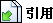引用網址：https://city.udn.com/forum/trackback.jsp?no=58536&aid=4584685